# ISEE Middle Level Math : How to find the points on a coordinate plane

## Example Questions

### Example Question #1 : How To Find The Points On A Coordinate Plane

Which of the following points will you find on the-axis?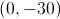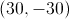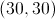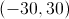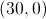Explanation:

A point is located on the-axis if and only if it has-coordinate (first coordinate) 0. Of the five choices, only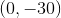fits that description.

### Example Question #3 : Coordinate Geometry

Which of the following points is on the-axis?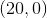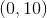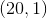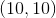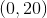Explanation:

A point is located on the-axis if and only if it has a-coordinate equal to zero.  So the answer is.

### Example Question #3 : How To Find The Points On A Coordinate Plane

A line segment on the coordinate plane has its endpoints at the points with coordinates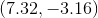and. Give the midpoint of the segment.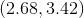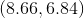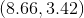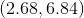Explanation:

The-coordinate of the midpoint can be found by dividing the sum of the-coordinates of the endpoints by 2: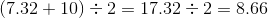The-coordinate of the midpoints is found similarly: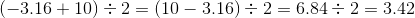### Example Question #4 : How To Find The Points On A Coordinate Plane

A line segment on the coordinate plane has its endpoints at the points with coordinates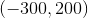and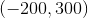. Give the coordinates of the midpoint of the segment.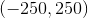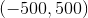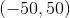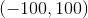Explanation:

The-coordinate of the midpoint can be found by dividing the sum of the-coordinates of the endpoints by 2: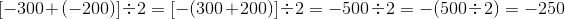The-coordinate of the midpoint is found similarly: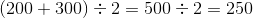.

The correct response is.

### Example Question #5 : How To Find The Points On A Coordinate Plane

A line segment on the coordinate plane has its endpoints at the points with coordinates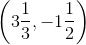and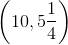.  Give the coordinates of the midpoint of the segment.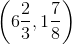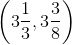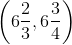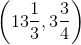Explanation:

The-coordinate of the midpoint is half the sum of the-coordinates of the endpoints: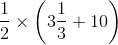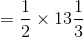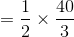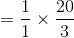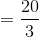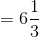The-coordinate of the midpoint is found similarly: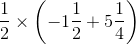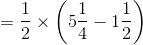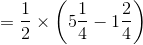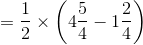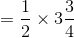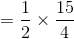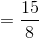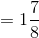The correct response is.

### Example Question #6 : How To Find The Points On A Coordinate Plane

Angela plotted points A through E on the coordinate plane shown below.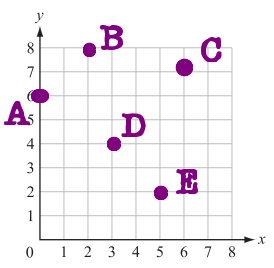Which point is located at the ordered pair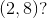Point D

Point C

Point A

Point E

Point B